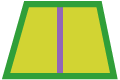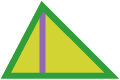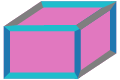# Calculation of areas and volumes of basic geometric shapes, Online

Practical application of mathematical formulas, where the change of each value directly changes the others.

## Cycle= π*
2
= 2*π*

## Rectangle=
*
= 2*
(
+
)

## Trapezoid= ((
*
)
*
)
/2
=
+
+
+

## Triangle=
(
*
)
/2
=
+
+

## Rectangular parallelepiped=
*
*
= 2*
((
*
)
+
(
*
)
+
(
*
))

= 4/3*π*
3
= 4*π*
2

## Cylinder= π*
2 *
= 2*π*
*
(
+
)

## Info

I chose the mathematical formulas that have a practical application so that in addition to educational purpose, they could also be practical tools for carpenters-craftsmen-engineers.

The above application is written in JavaScript programming language and designed to be easy to use.

It belongs to the application family Simple & Nice Apps, snapps, with the following facebook page.